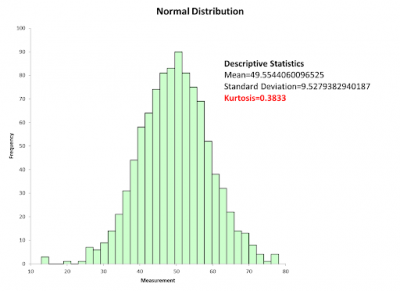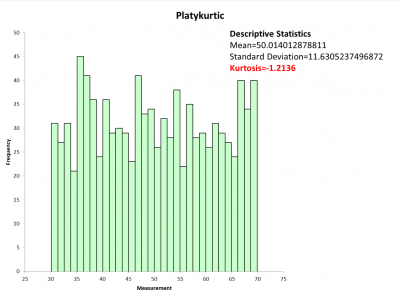Many statistical tools have an underlying assumption your data will be normal. But, what if your data distribution looks different from the standard normal curve, what does that mean?

## Overview: What is a platykurtic distribution?

Kurtosis is one of the key measures used for describing the shape of the normal distribution. Kurtosis is a measure of the height of your data distribution and the length of the tails relative to normal distribution.  Kurtosis refers to the tailedness of the distribution rather than the peakedness. The value for kurtosis of a normal distribution is 3, and the shape is referred to as mesokurtic. Kurtosis for a standard normal distribution will be zero.

Leptokurtic describes a distribution where the value for kurtosis is greater than 3 when compared to a normal distribution and a value larger than zero when compared to a standard normal distribution.

Platykuritc describes a distribution where the center of the curve will be shorter than a normal or mesokurtic distribution, and the tails will be lighter with fewer values in the tail. Below is a graph comparing a normal distribution with a platykurtic and leptokurtic one. Note the lower tails for the platykurtic distribution.

## An industry example of a platykurtic distribution

The company Black Belt (BB) was reviewing the graphical output of her data to see if it was normally distributed so she could easily do the needed statistical calculations. Unfortunately, the shape of her data was platykurtic.

Her interpretation was the data had less outliers than a normal distribution due to the thinner and shorter tails. She was concerned the outliers might impact the validity of her analysis.

Below is a graph of some normally distributed process data plus a graph of data which appears to be platykurtic. Note the value of kurtosis with the normal distribution is close to 0 and the platykurtic value is negative.### 1. How would you describe a platykurtic distribution?

A platykurtic distribution has a lower peak and wider bell shape. It will also have longer and shorter tails than a normal distribution. A high negative value of kurtosis will indicate the platykurtic distribution has less of the values located in the tails of the distribution than around the center or average.

### 2. Where does the term platykurtic come from?

Kurtosis is derived from a transliteration of the Greek word kurtos, meaning curved or arched. Kurtic stems from that. Platy also comes from Greek and means broad In terms of shape. A platykurtic distribution has thinner tails.

### 3. How do I know if my distribution is platykurtic?

Visually, you can compare it to a theoretical normal distribution for your parameters. If the peak of your distribution looks lower than the normal distribution and the tails lower and longer then visually you might claim the distribution is platykurtic. Statistically, if the value for the kurtosis of your distribution is less than 3 or less than zero for a standard normal distribution then your distribution can be classified as platykurtic.